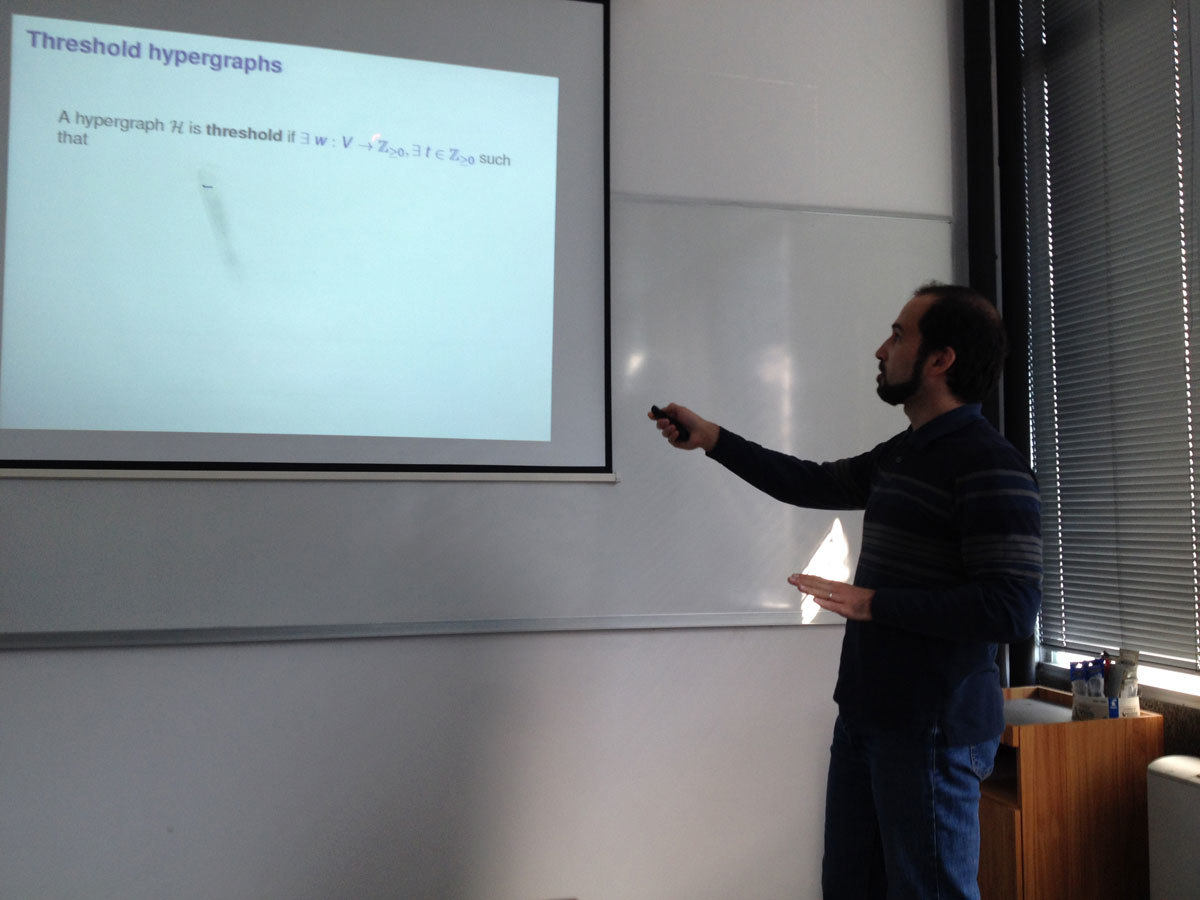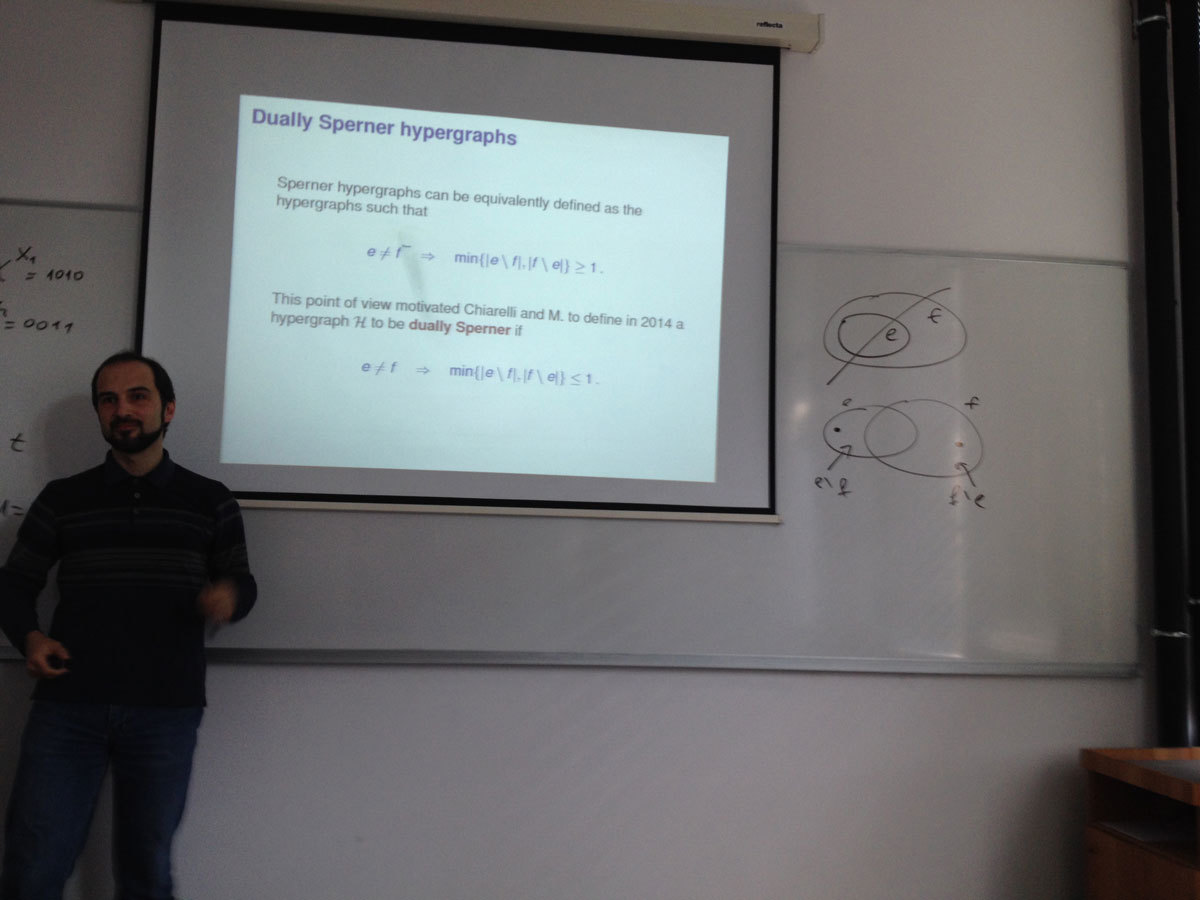Univerza na Primorskem Fakulteta za matematiko, naravoslovje in informacijske tehnologije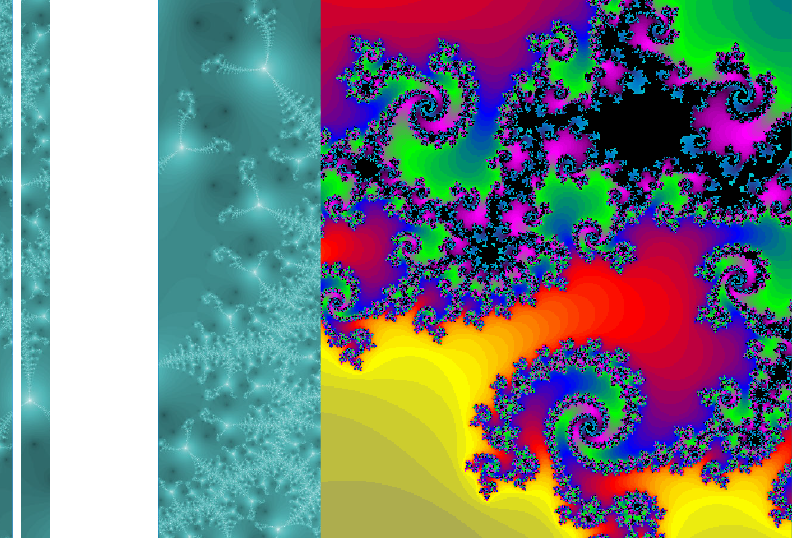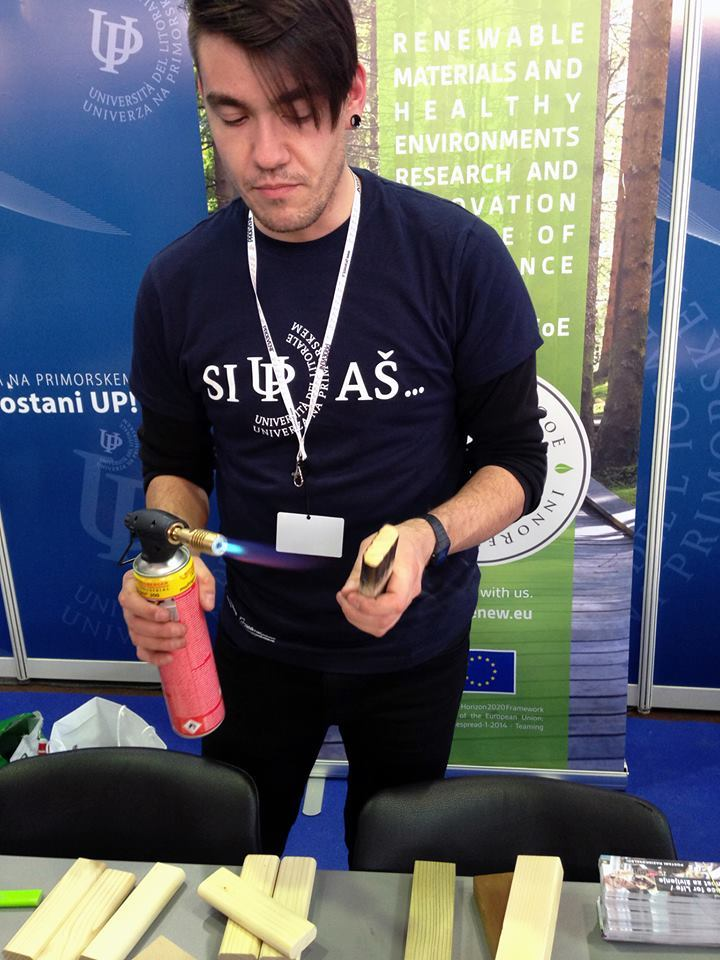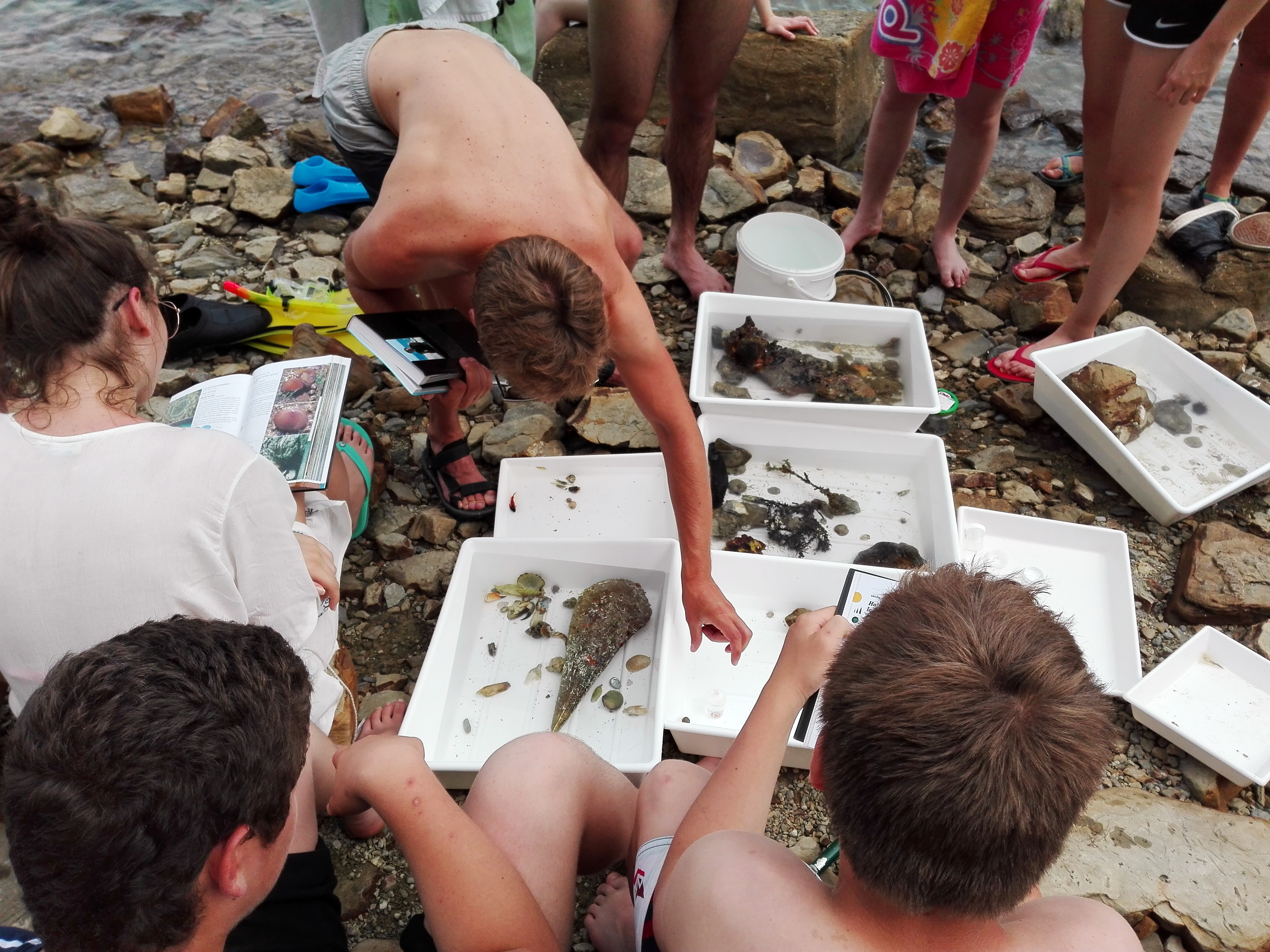SI | EN# Raziskovalni matematični seminar - Arhiv

 2021 2020 2019 2018 2017 2016 2015 2014 2013 2012 2011 2010
 1 2 3 4 5 6 7 8 9 10 11 12
Datum in ura / Date and time: 30.11.15
(10:00-11:00)
Predavalnica / Location: FAMNIT-POSTA
Predavatelj / Lecturer: István Kovács (UP IAM, UP FAMNIT)
Naslov / Title: Integral automorphisms of affine spaces over finite fields
Vsebina / Abstract:

Let \$GF(q)\$ denote the finite field with \$q\$ elements, and let \$S\$ denote the set of all square elements of \$GF(q)\$. The norm \$N\$ of a point \$x=(x_1,\ldots,x_n)\$ of the affine space \$AG(n,q)\$ is defined by \$N(x) = x_1^2+\cdots+x_n^2,\$ and two points \$x\$ and \$y\$ are said to be at integral distance if \$N(x-y)\$ is in \$S\$. An integral  automorphism of \$AG(n,q)\$ is a permutation of the point set which  preserves integral distances. In this talk I present some recent results on integral automorphisms. The talk is based on a joint work with Klavdija Kutnar, J\'anos Ruff and Tam\'as Sz\H onyi.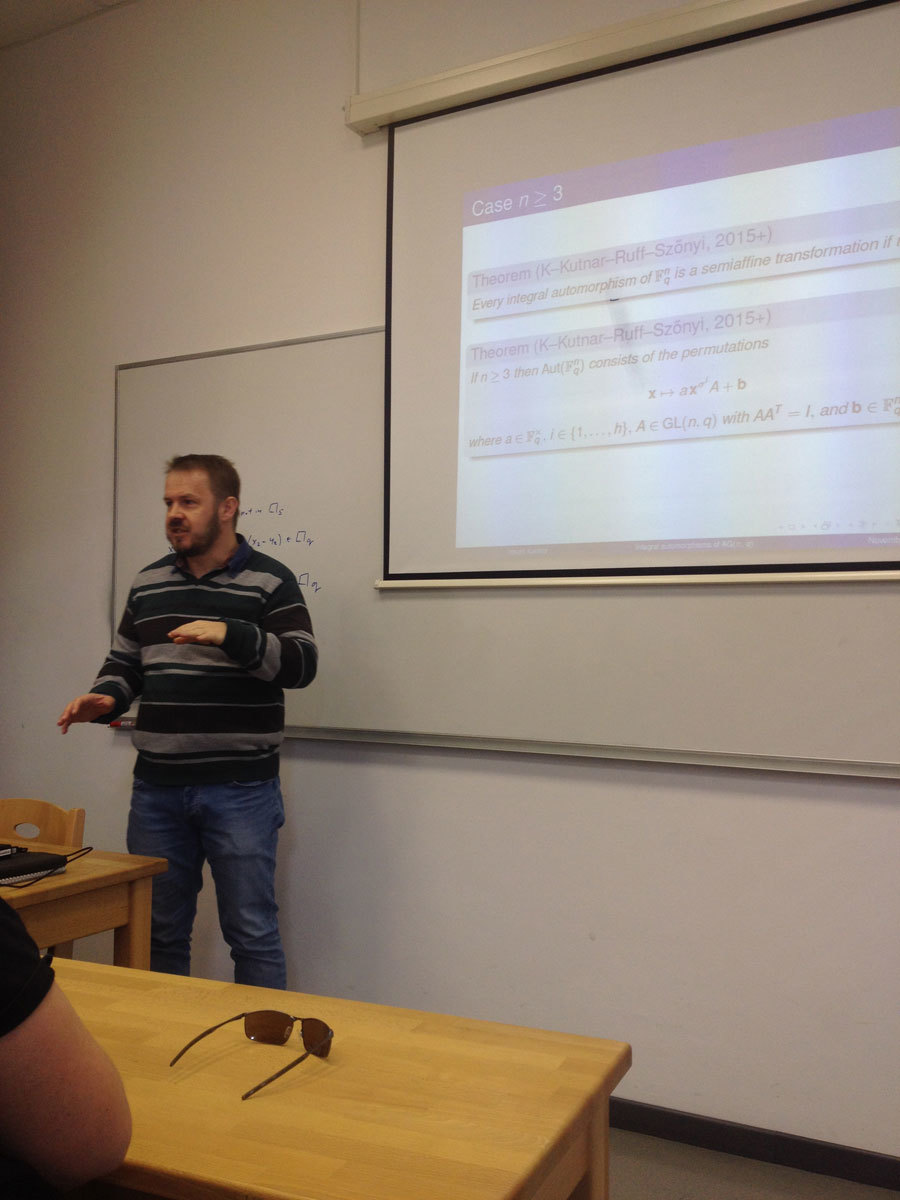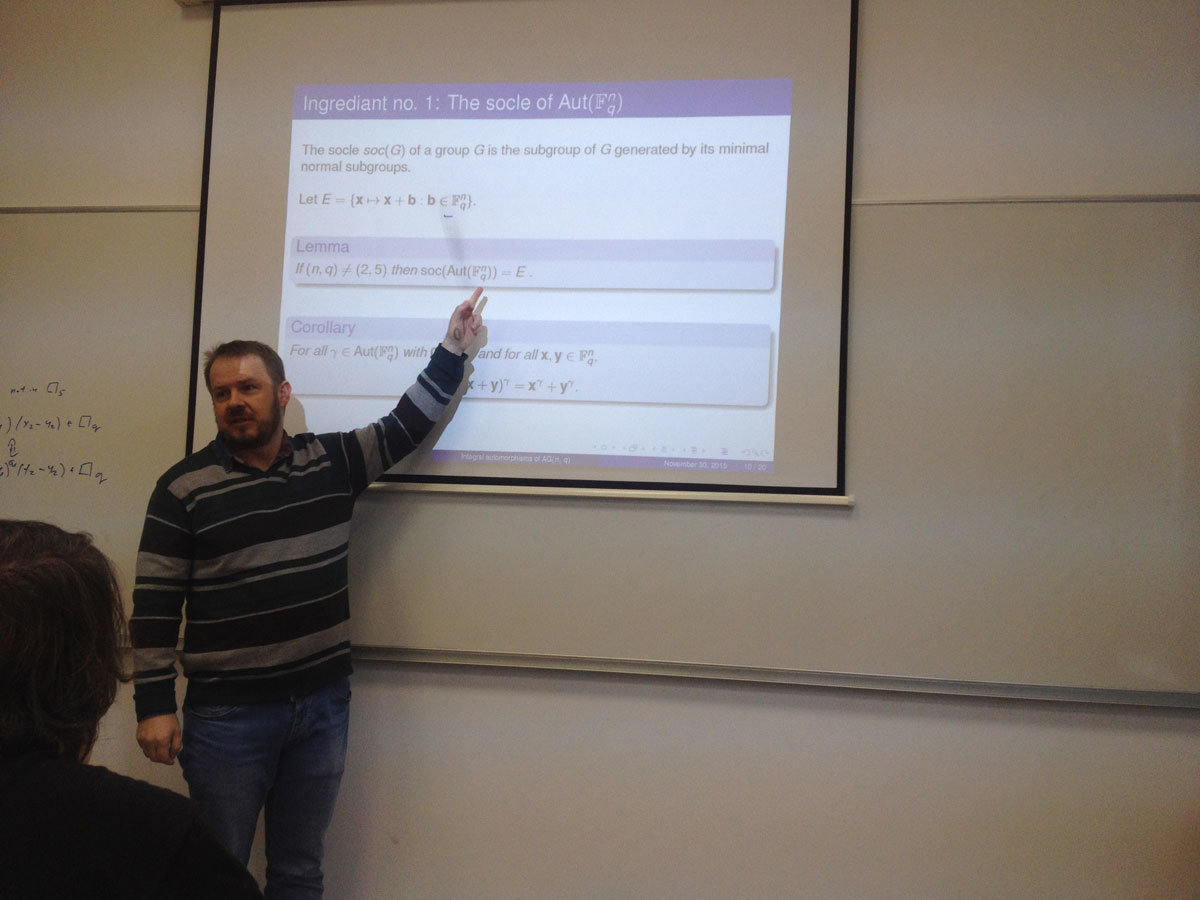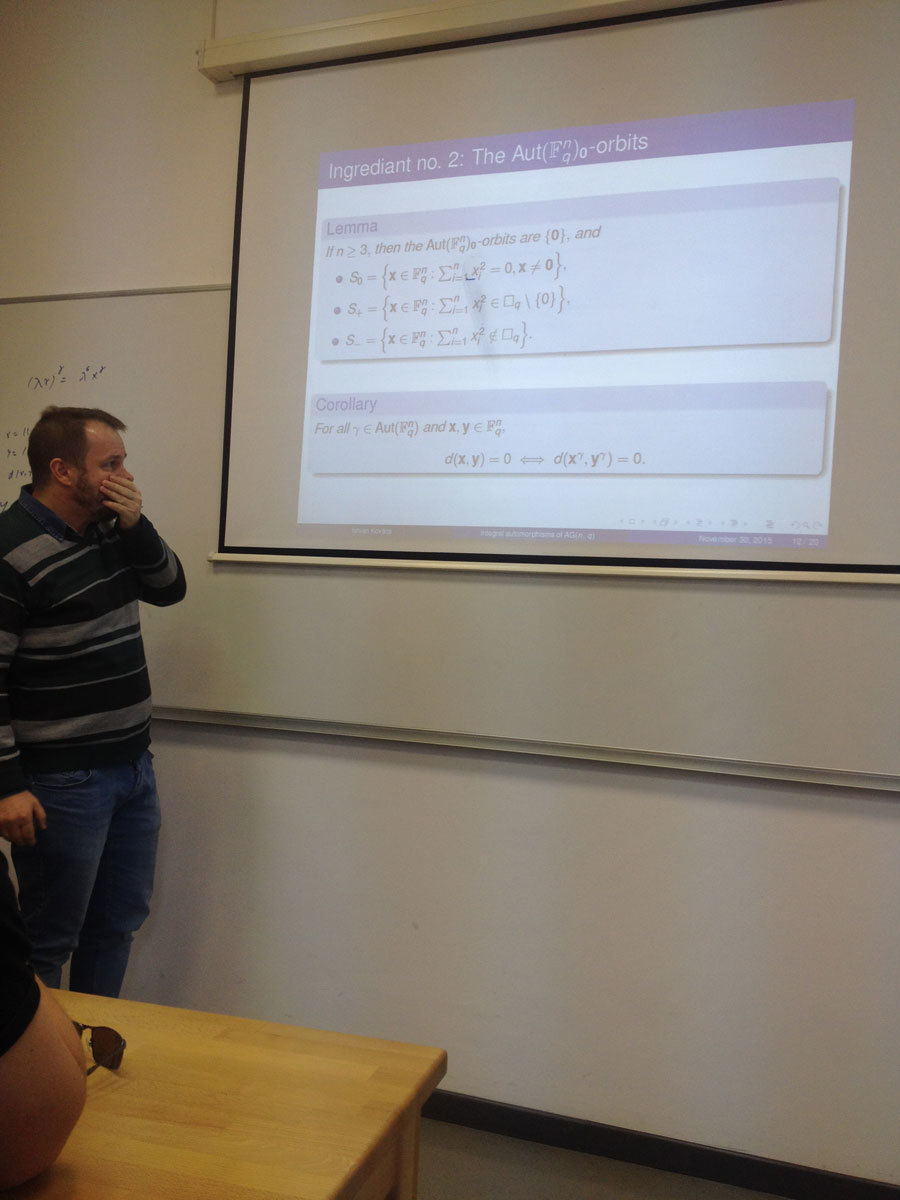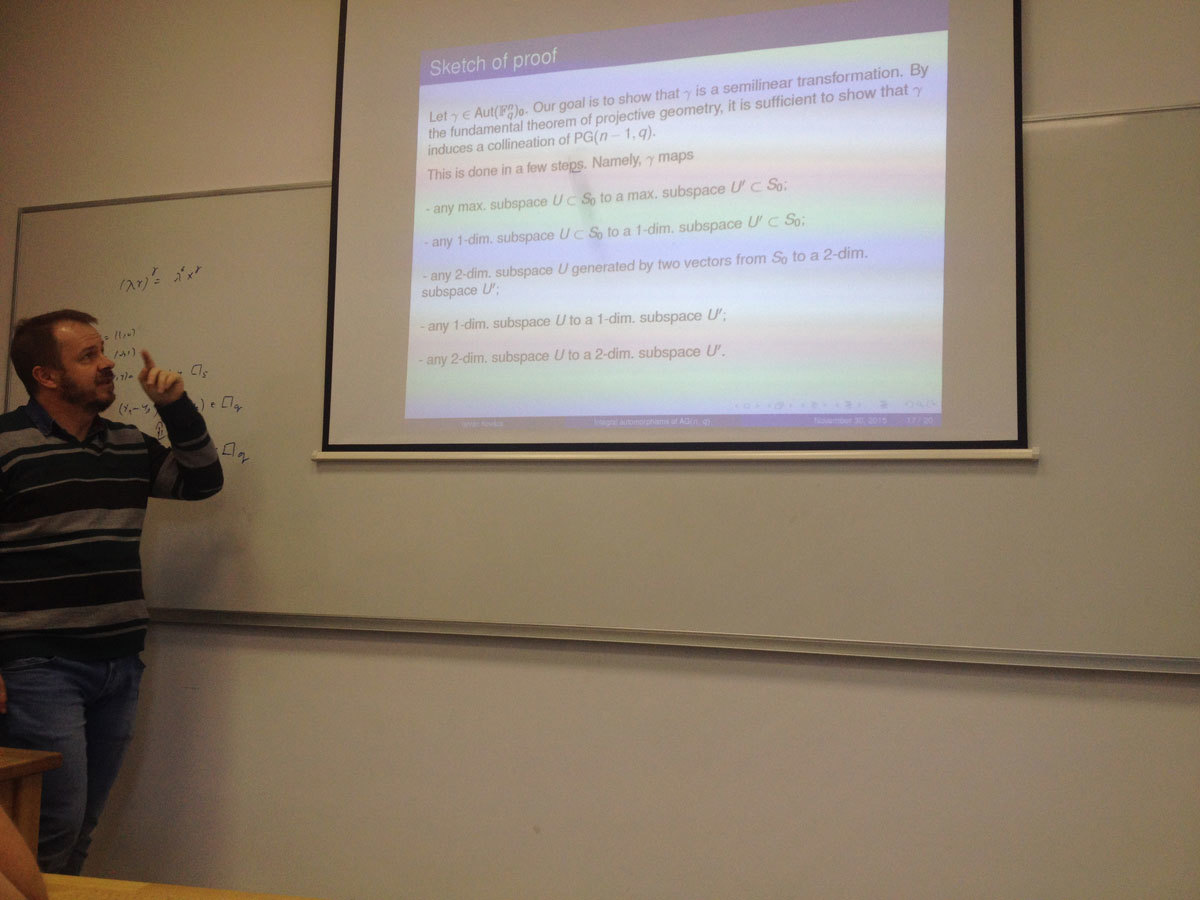Datum in ura / Date and time: 23.11.15
(10:00-11:00)
Predavalnica / Location: FAMNIT-POŠTA
Predavatelj / Lecturer: Robert Jajcay (UP FAMNIT and Comenius University (Slovakia))
Naslov / Title: k-regular families of automorphisms and generalized Cayley graphs
Vsebina / Abstract:

Abstract: A family F of automorphisms of a vertex-transitive graph G is said to be k-regular if for every pair u,v of vertices of G there exist k automorphisms in F mapping u to v. Every vertex-transitive graph admits a k-regular family for some positive k, and quasi-Cayley graphs are vertex-transitive graphs admitting a 1-regular family of automorphisms. We investigate the class of merged Johnson graphs with regard to the parameter k, classify merged Johnson graphs that are Cayley, and relate this topic to that of the existence of a uniform routing.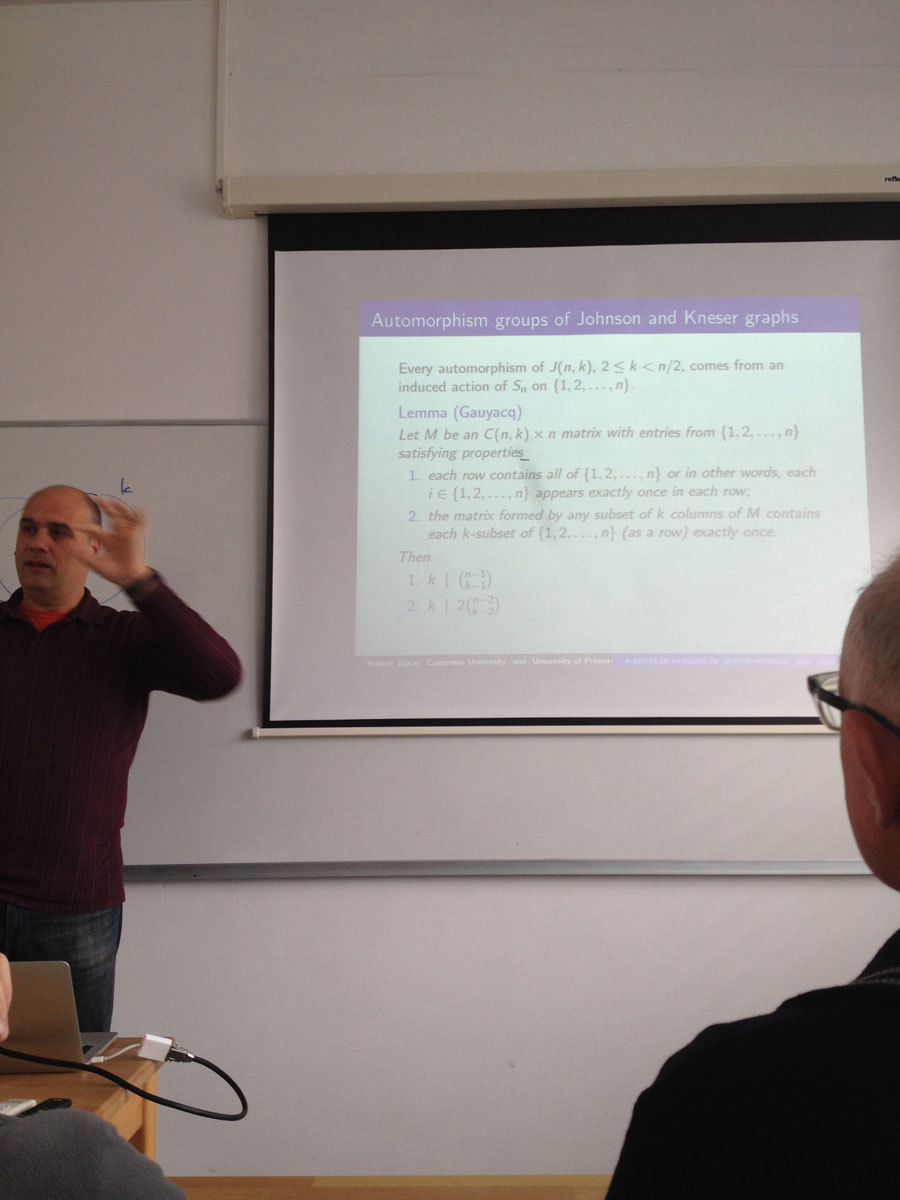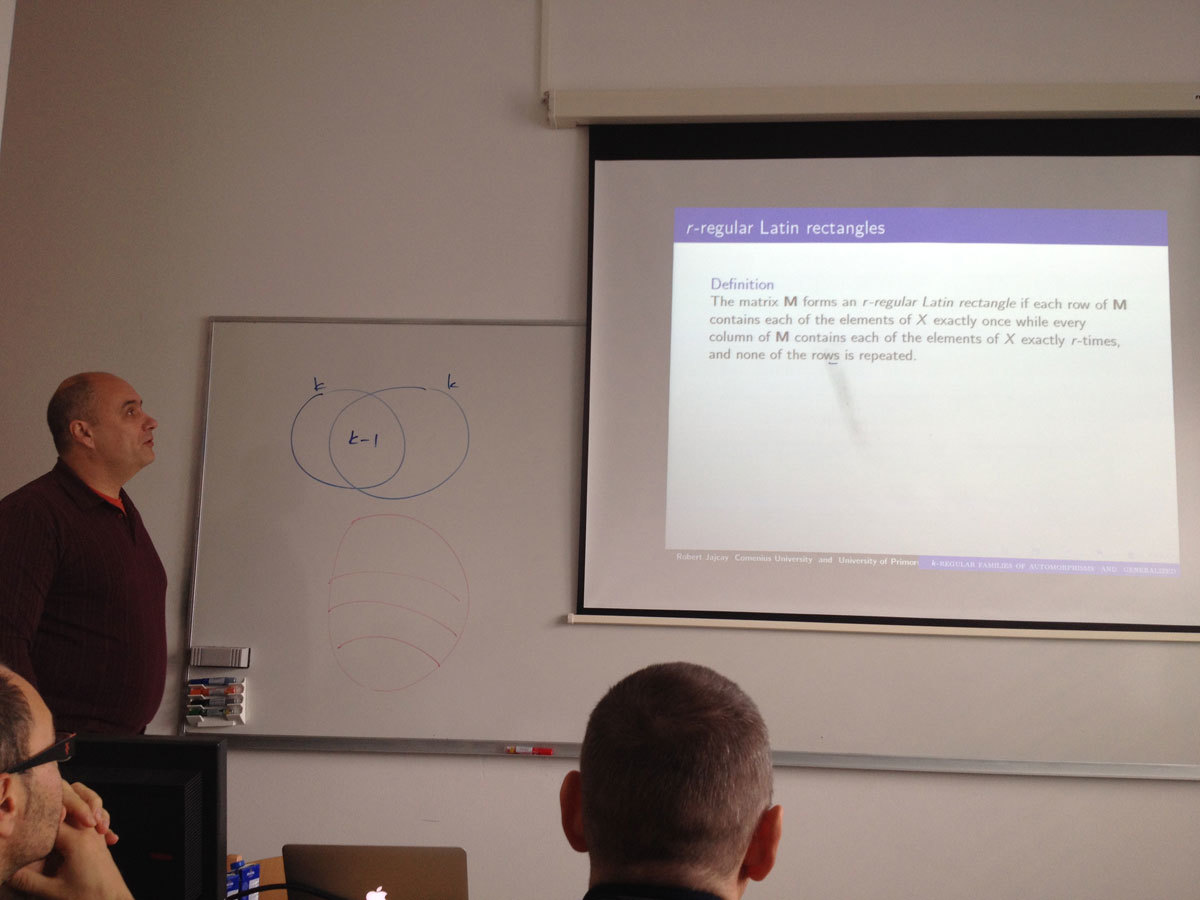Datum in ura / Date and time: 16.11.15
(10:00-11:00)
Predavalnica / Location: FAMNIT-POSTA
Predavatelj / Lecturer: Edin Husić
Naslov / Title: Submodular functions
Vsebina / Abstract:

In this talk we will give an overview of various aspects of submodular set functions. Several examples of submodular functions will be presented, including modular functions, polymatroid functions, and objective functions of various optimization problems, for example those related to facility location problems and to cuts in graphs.

The problem of minimization and maximization of submodular functions, and the submodular set cover problem will be discussed. Two approximation algorithms will be described: one for the problem of maximizing a given submodular function under a cardinality constraint due to Nemauser et al. and one for the unconstrained submodular maximization due to Buchbinder et al. More precisely, we will present a greedy algorithm for maximizing a submodular function under a cardinality constraint, one deterministic algorithm and two randomized algorithms with approximation factors 1/3, 1/4 and 1/2, respectively, for solving the uncostrained submodular maximization problem.

Finally, a new proof of the theorem due to Boros et al. stating that every hypergraph has an exact polymatroid separator will be given.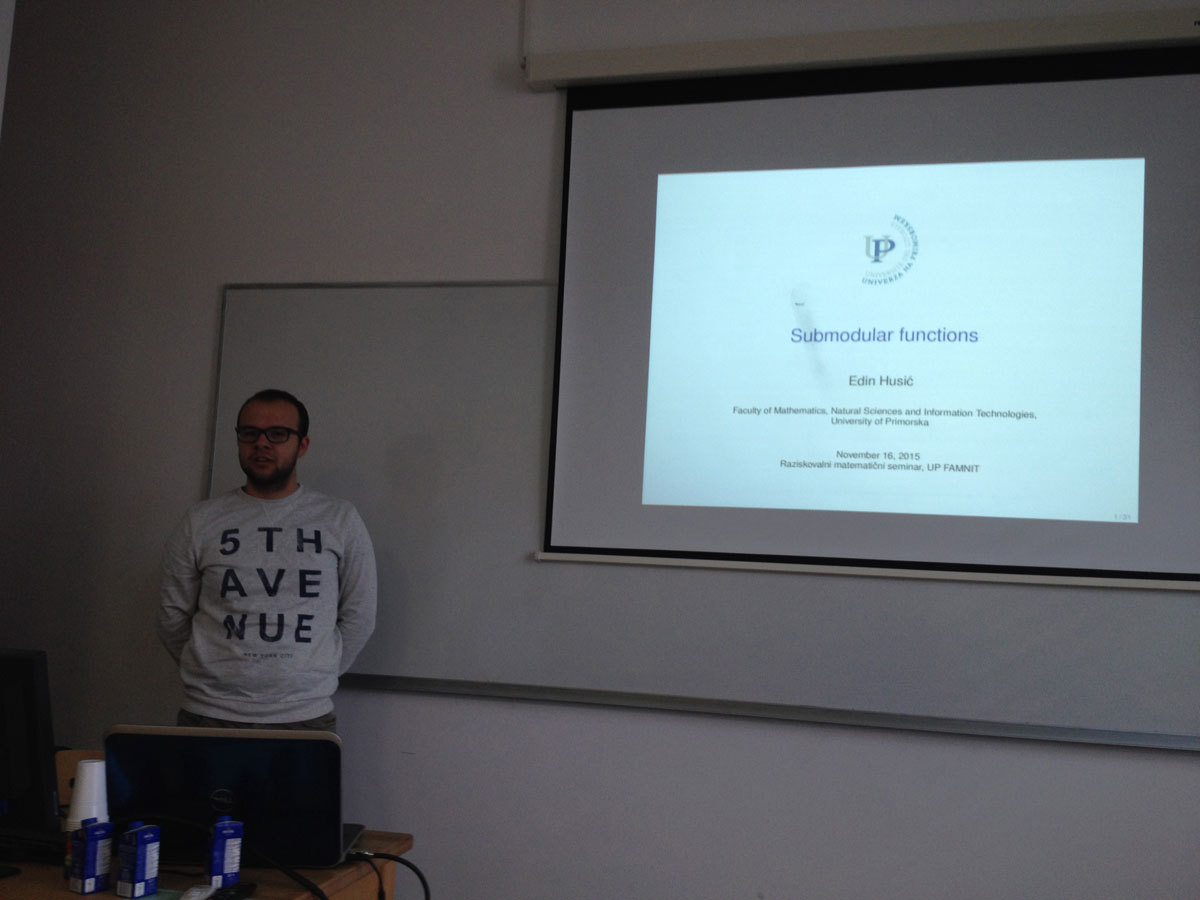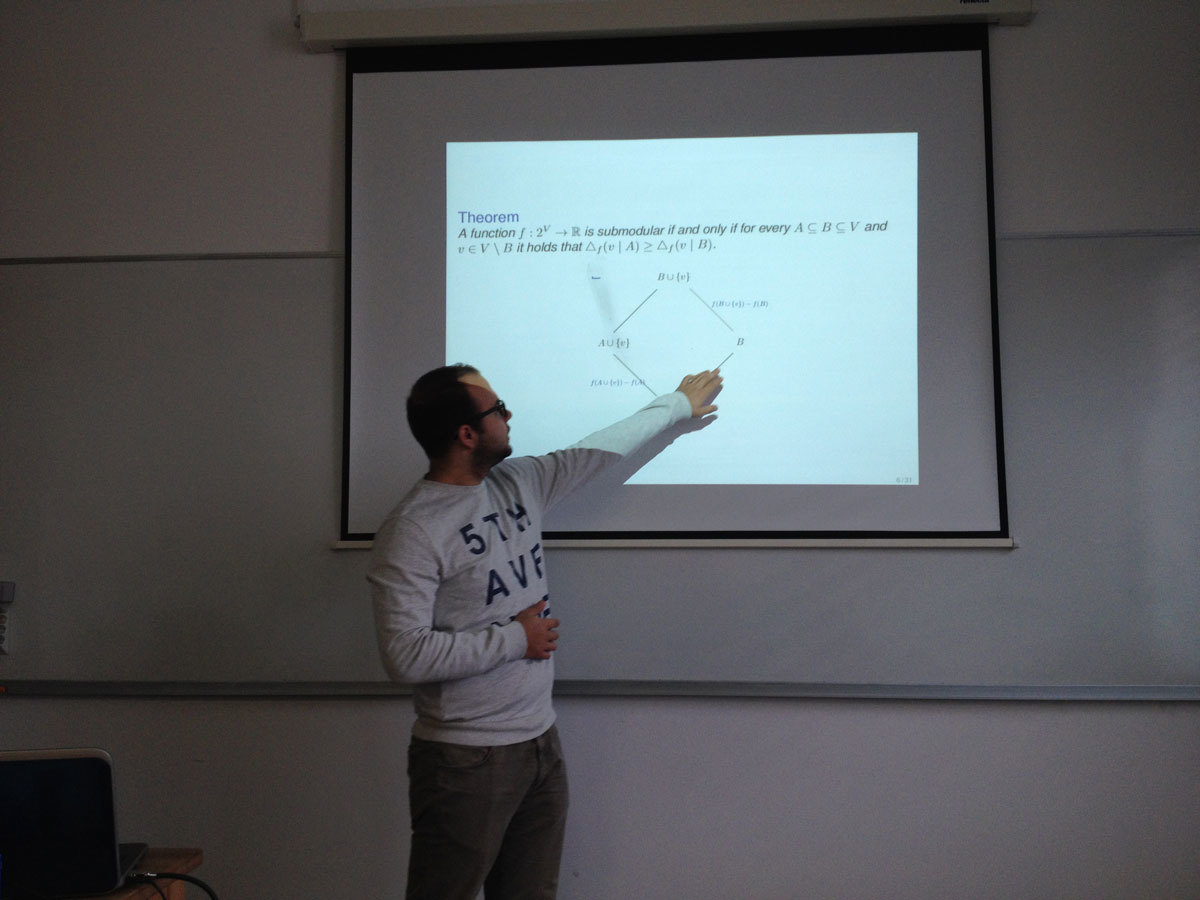Datum in ura / Date and time: 9.11.15
(10:00-11:00)
Predavalnica / Location: FAMNIT-POSTA
Predavatelj / Lecturer: Martin Milanič (UP IAM, UP FAMNIT)
Naslov / Title: 1-Sperner hypergraphs and new characterizations of threshold graphs
Vsebina / Abstract:

A hypergraph is a pair (V,E) such that V is a finite set and E is a set of subsets of V (members of E are called hyperedges). We introduce a new class of hypergraphs, the class of 1-Sperner hypergraphs. A hypergraph H is said to be 1-Sperner if every two distinct hyperedges e,f of H satisfy min { | e \ f | ,| f \ e | } = 1.

We prove a decomposition theorem for 1-Sperner hypergraphs and examine several of its consequences, including bounds on the size of 1-Sperner hypergraphs and a new, constructive proof of the fact that every 1-Sperner hypergraph is threshold. (A hypergraph H = (V,E) is said to be threshold if there exist a non-negative vertex weight function w and a non-negative threshold t such that for every subset X of V, we have w(X) >= t if and only if X contains a hyperedge.)

A hypergraph is said to be Sperner (or: a clutter) if no hyperedge is contained in another one. Given a graph G, the clique hypergraph of G is the hypergraph C(G) with vertex set V(G) in which the hyperedges are exactly the maximal cliques of G. The clique hypergraphs of graphs are known to be exactly those Sperner hypergraphs H that are also normal (or: conformal), that is, for every subset X of V(H) such that every pair of elements in X is contained in a hyperedge, there exists a hyperedge containing X.

We show that within the class of normal Sperner hypergraphs, the (generally properly nested) classes of 1-Sperner hypergraphs, of threshold hypergraphs, and of 2-asummable hypergraphs coincide. This yields new characterizations of the class of threshold graphs.

The talk is based on joint work with Endre Boros and Vladimir Gurvich (both at Rutgers University).

Preprint is available at: http://arxiv.org/abs/1510.02438

Slides of talk are here.